Скачать презентацию Example 1 A Applying the Perpendicular Bisector Theorem

bb8e92a8748069115c151af4f17f81f1.ppt

• Количество слайдов: 60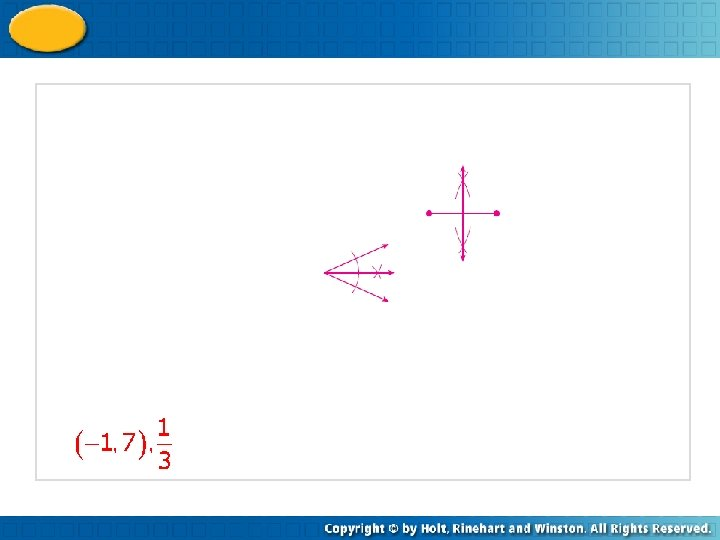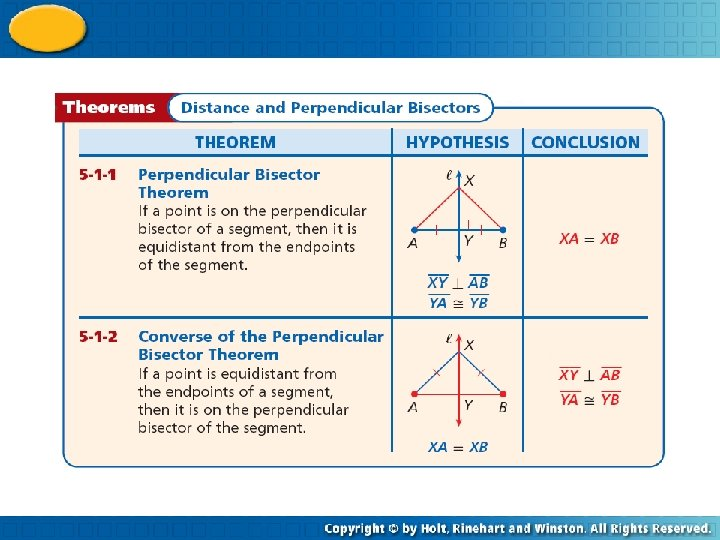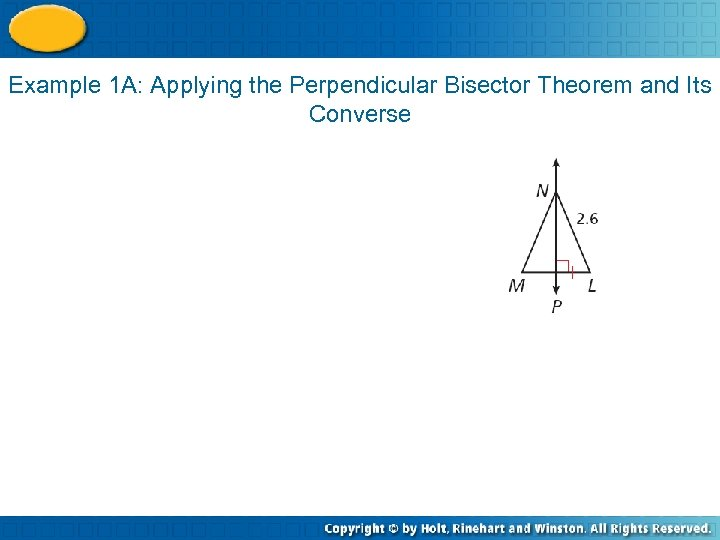Example 1 A: Applying the Perpendicular Bisector Theorem and Its Converse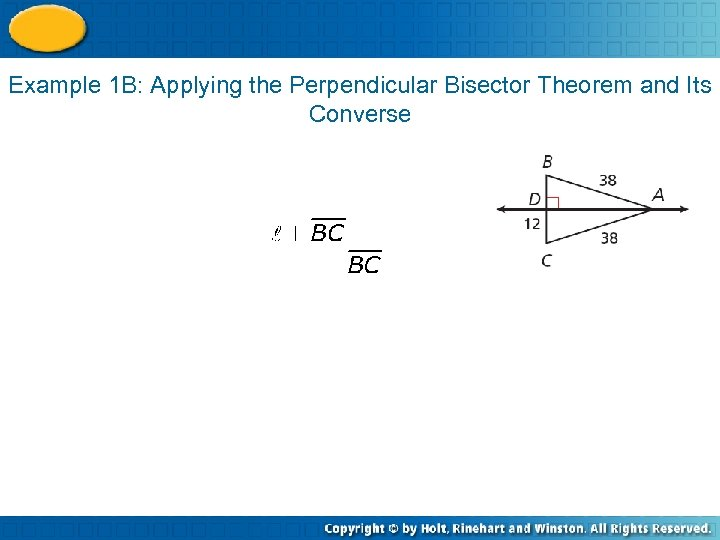Example 1 B: Applying the Perpendicular Bisector Theorem and Its Converse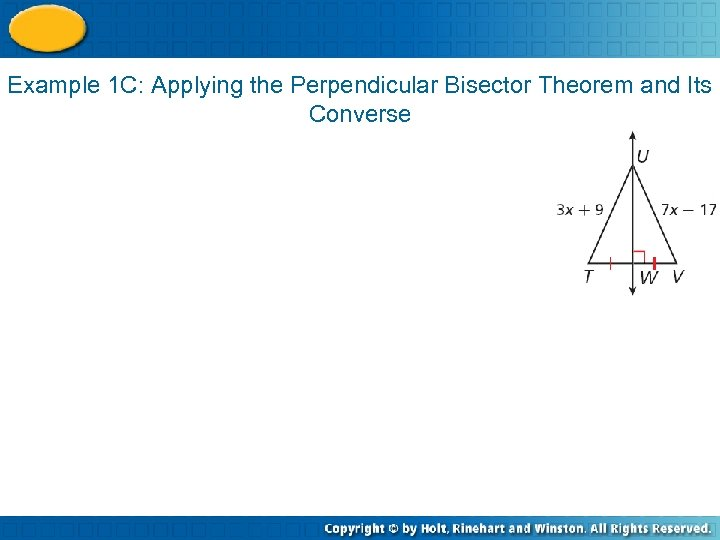Example 1 C: Applying the Perpendicular Bisector Theorem and Its Converse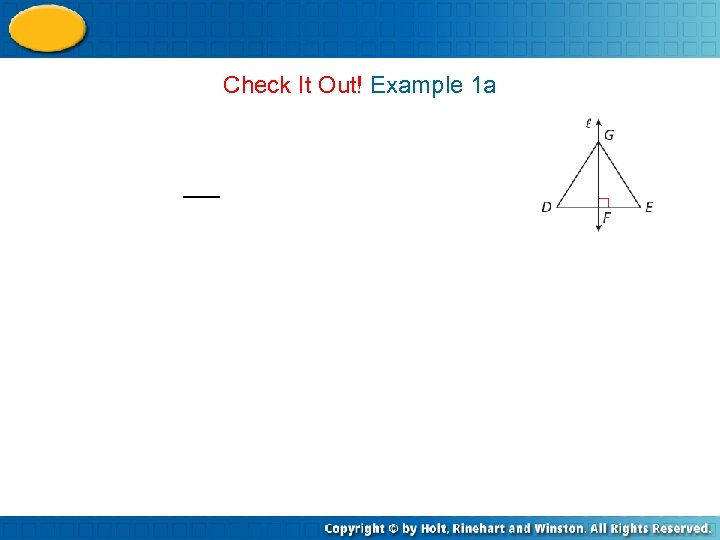Check It Out! Example 1 a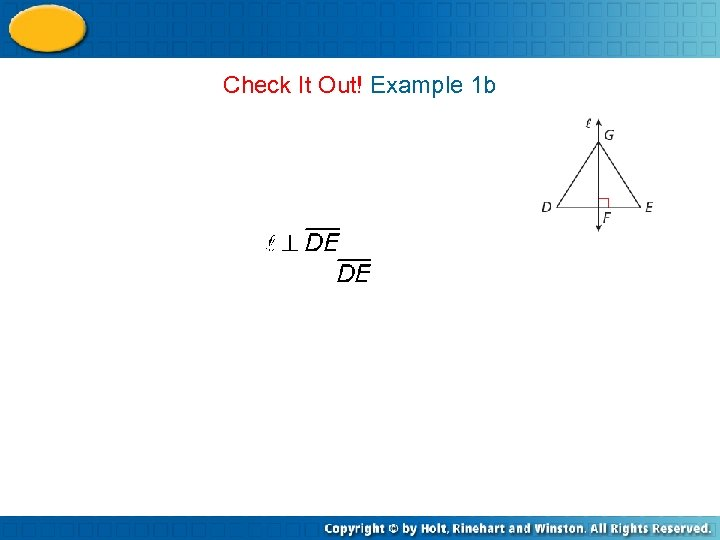Check It Out! Example 1 b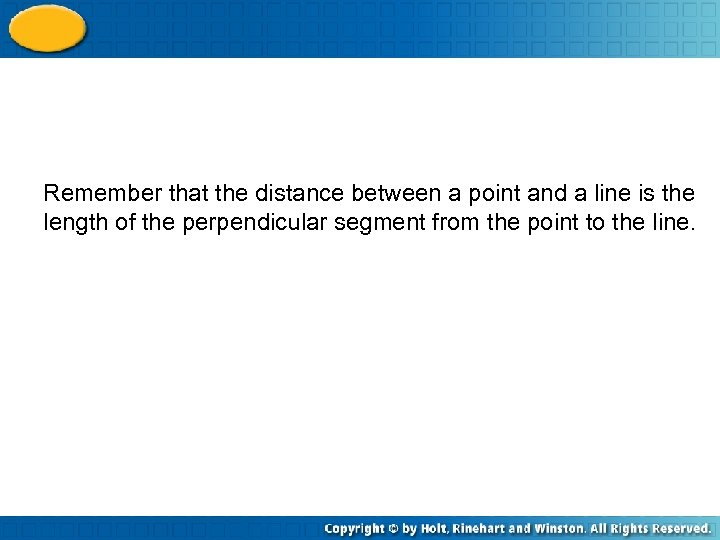Remember that the distance between a point and a line is the length of the perpendicular segment from the point to the line.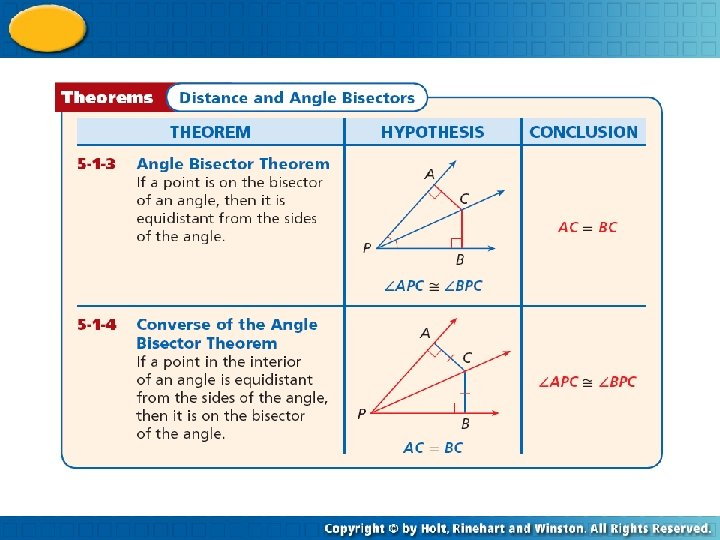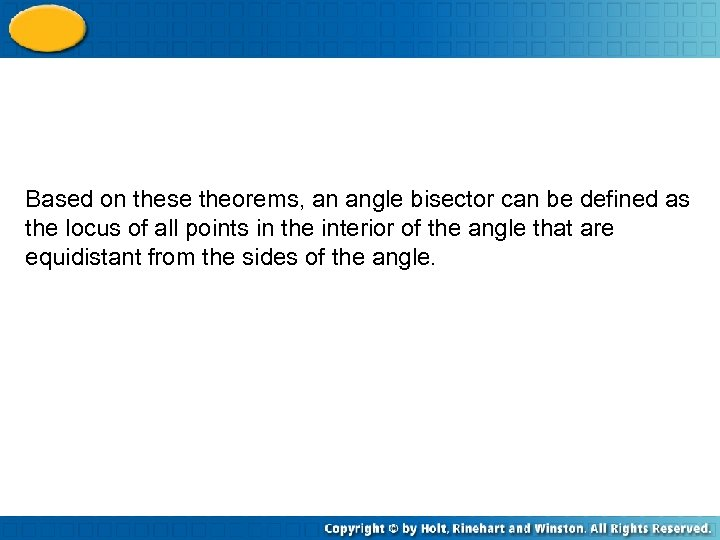Based on these theorems, an angle bisector can be defined as the locus of all points in the interior of the angle that are equidistant from the sides of the angle.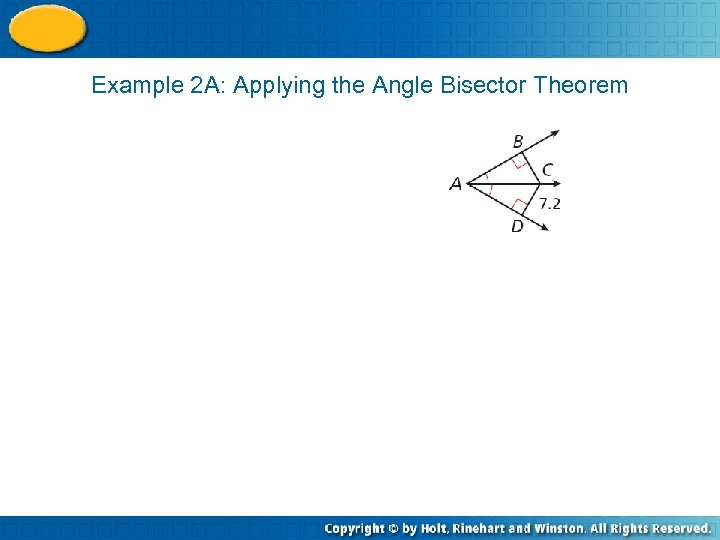Example 2 A: Applying the Angle Bisector Theorem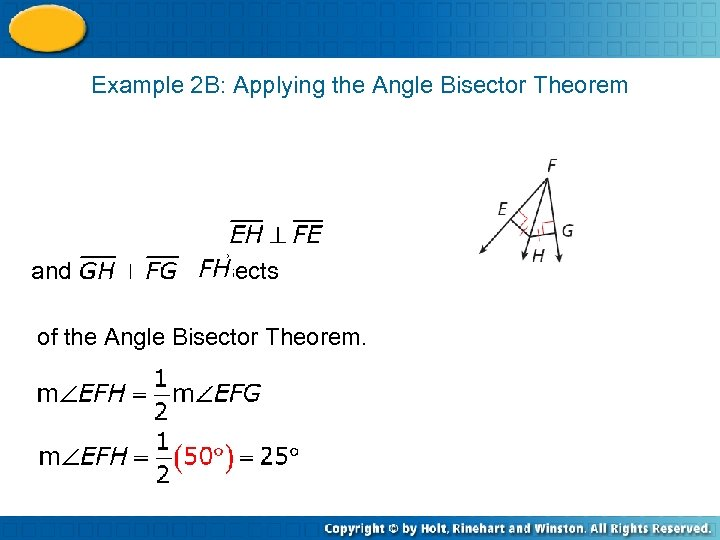Example 2 B: Applying the Angle Bisector Theorem and , bisects of the Angle Bisector Theorem.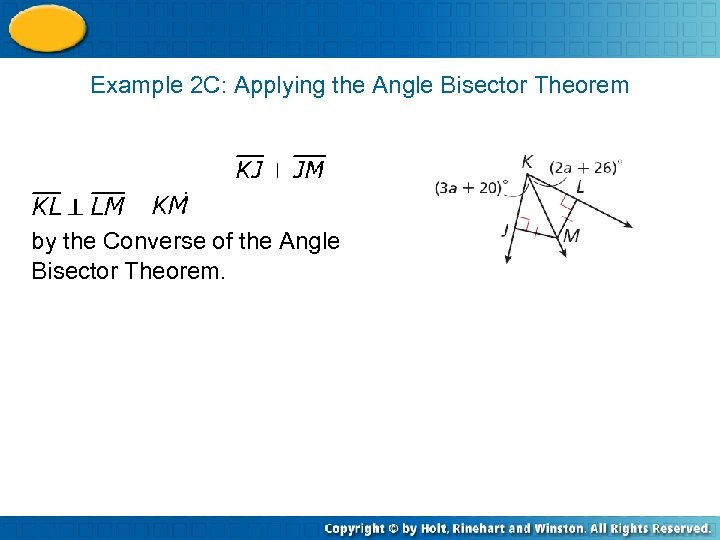Example 2 C: Applying the Angle Bisector Theorem by the Converse of the Angle Bisector Theorem.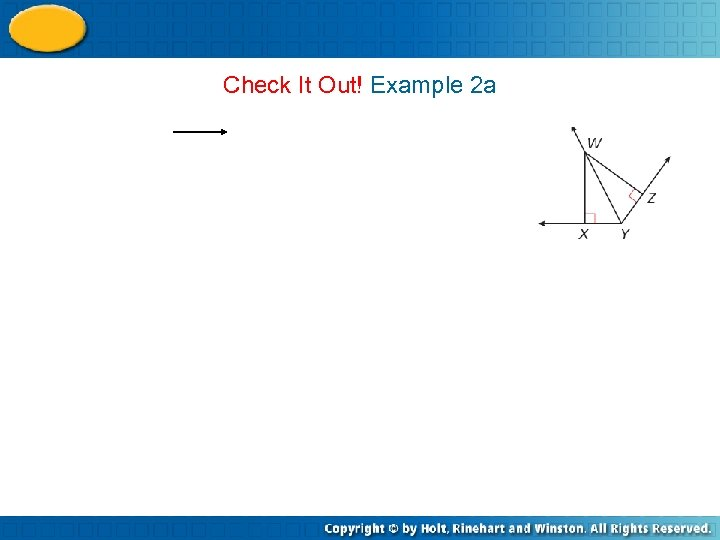Check It Out! Example 2 a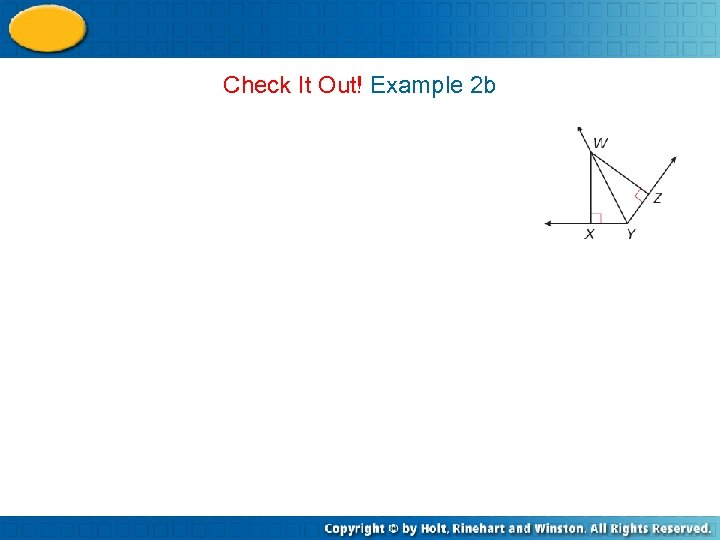Check It Out! Example 2 b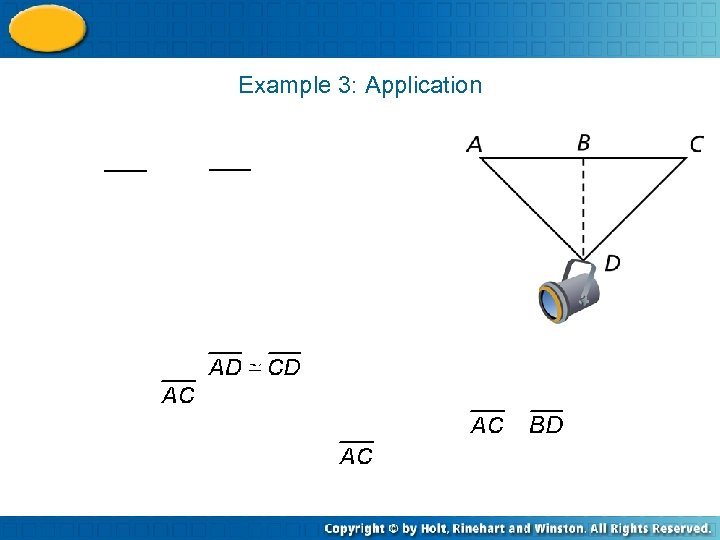Example 3: Application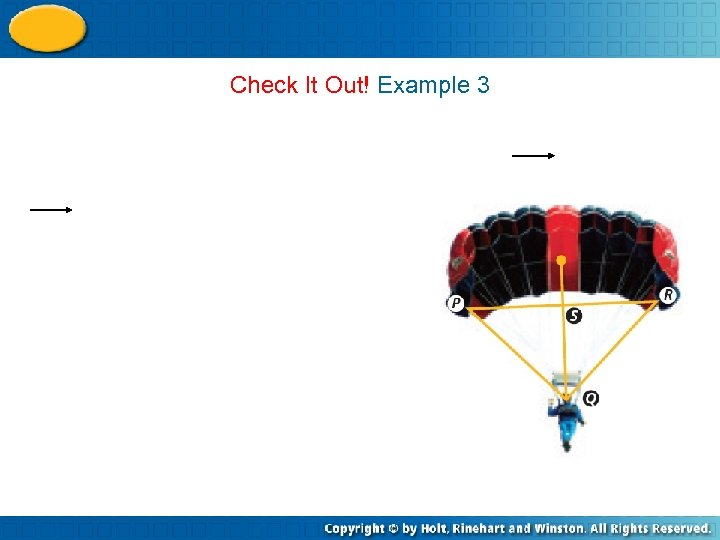Check It Out! Example 3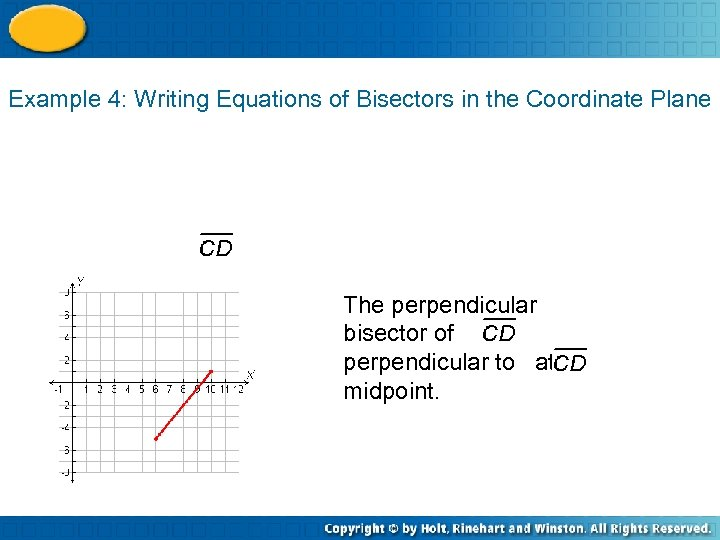Example 4: Writing Equations of Bisectors in the Coordinate Plane The perpendicular bisector of is perpendicular to at its midpoint.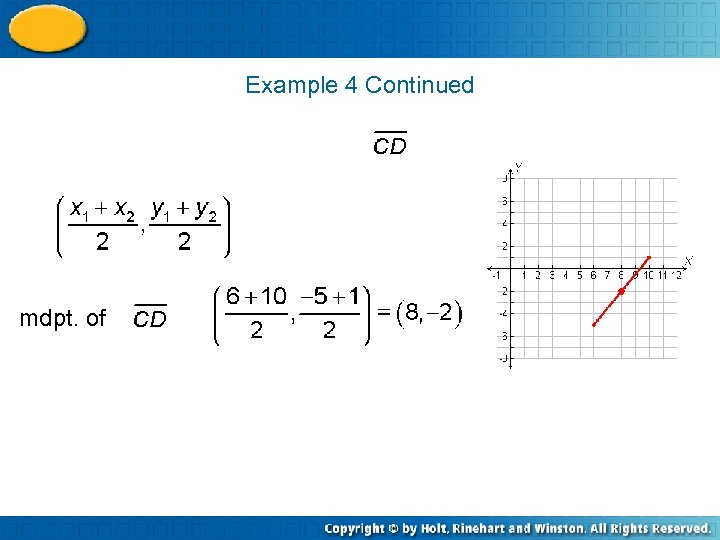Example 4 Continued mdpt. of =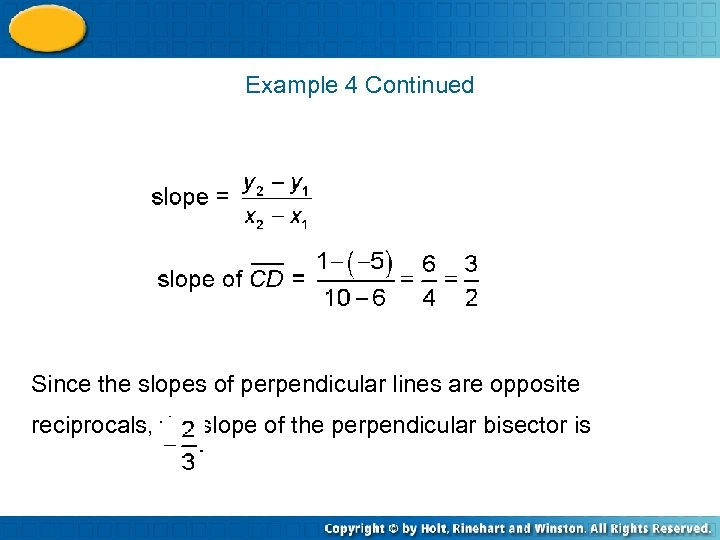Example 4 Continued Since the slopes of perpendicular lines are opposite reciprocals, the slope of the perpendicular bisector is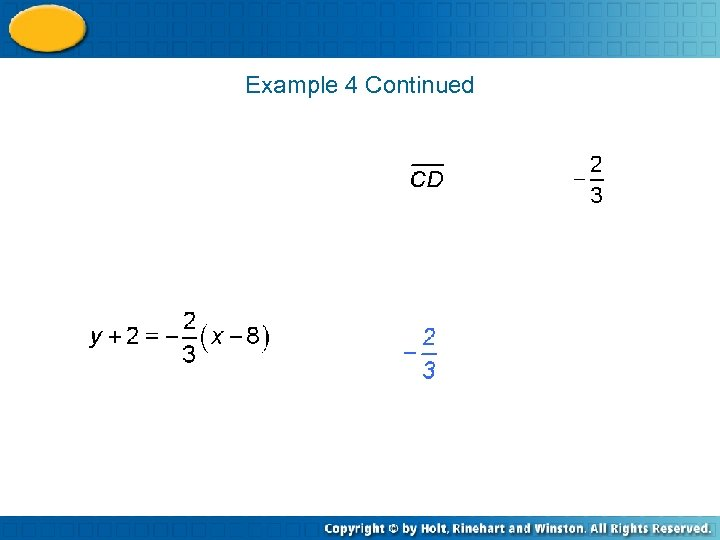Example 4 Continued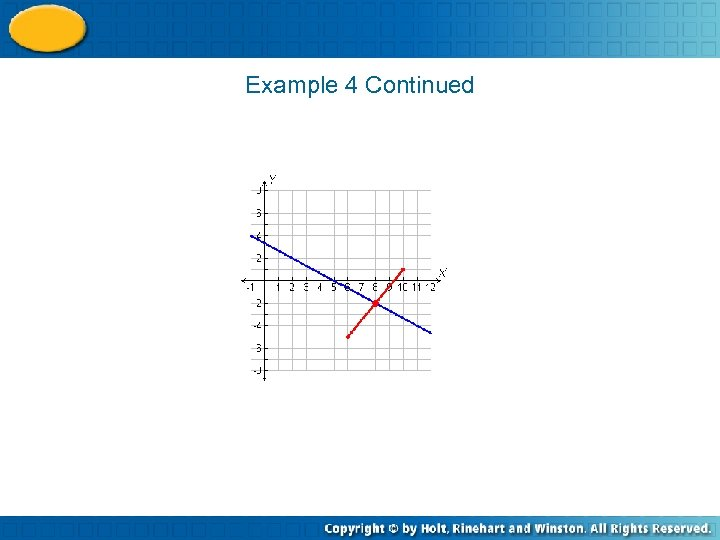Example 4 Continued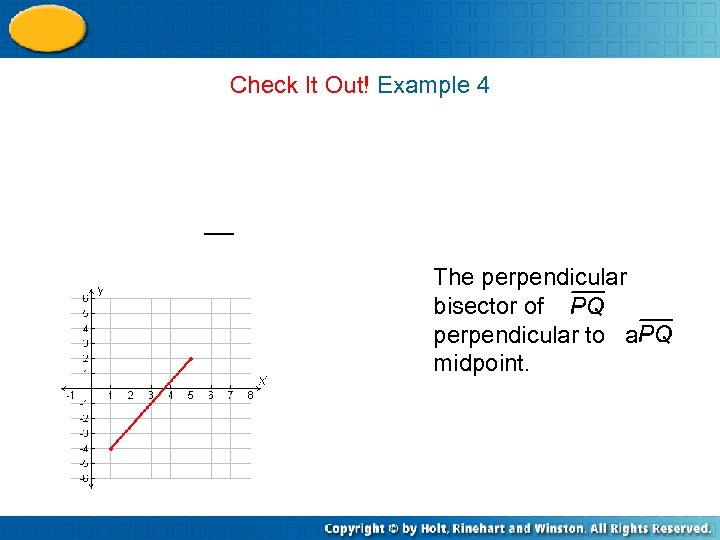Check It Out! Example 4 The perpendicular bisector of is perpendicular to at its midpoint.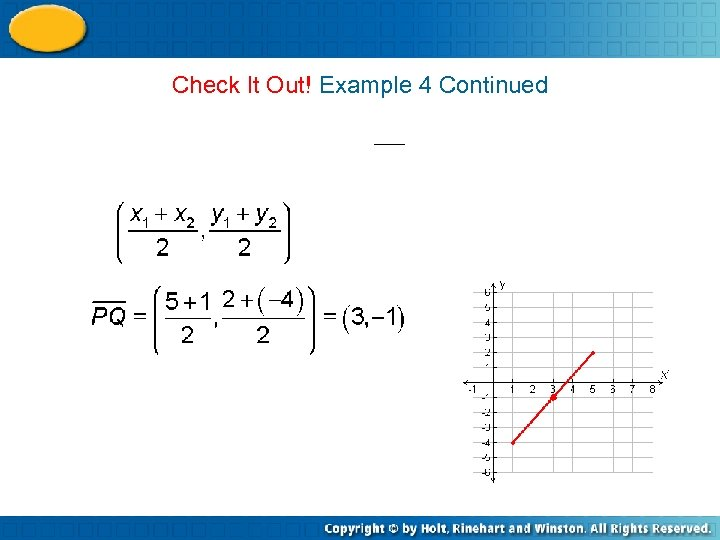Check It Out! Example 4 Continued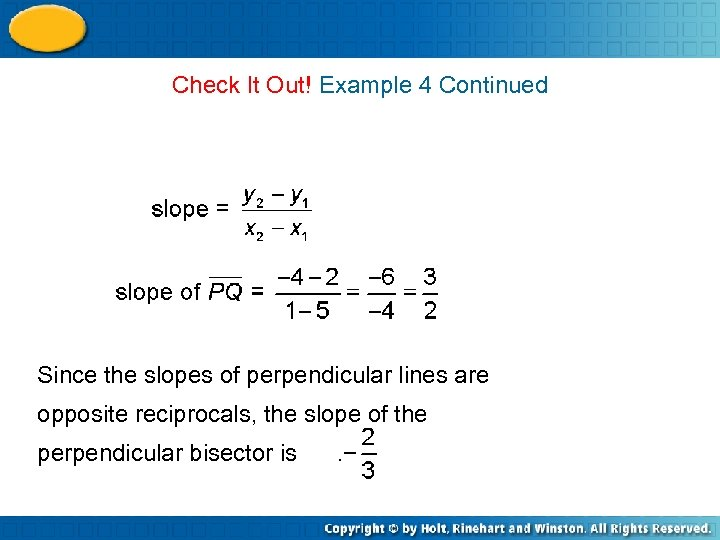Check It Out! Example 4 Continued Since the slopes of perpendicular lines are opposite reciprocals, the slope of the perpendicular bisector is .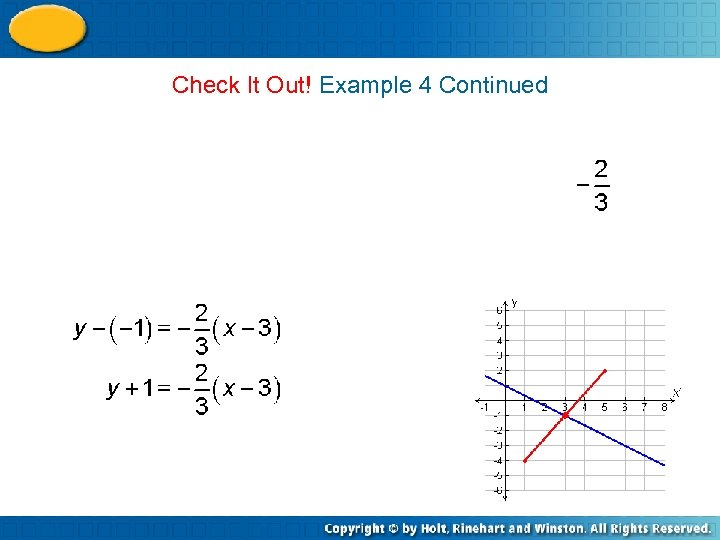Check It Out! Example 4 Continued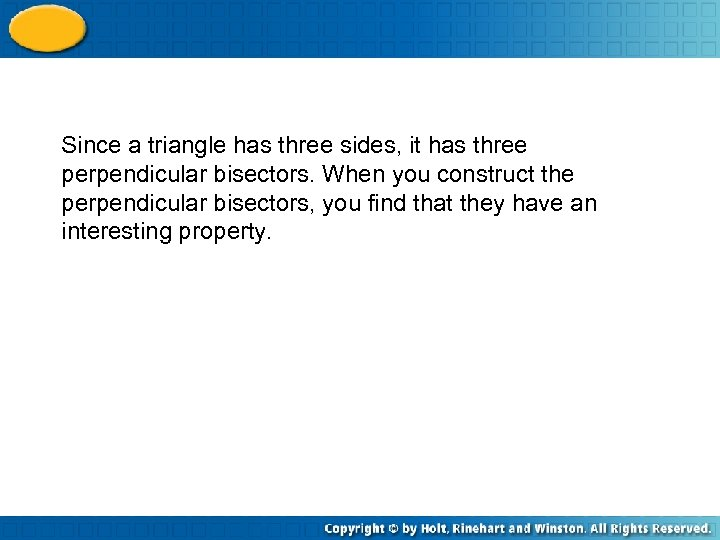Since a triangle has three sides, it has three perpendicular bisectors. When you construct the perpendicular bisectors, you find that they have an interesting property.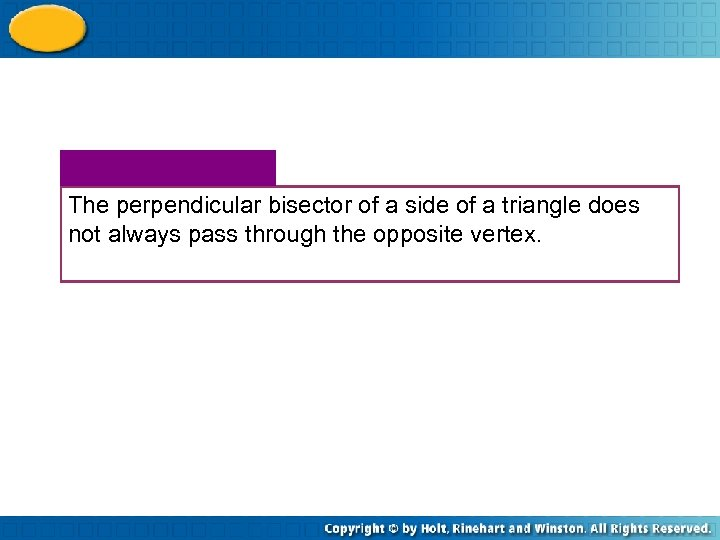The perpendicular bisector of a side of a triangle does not always pass through the opposite vertex.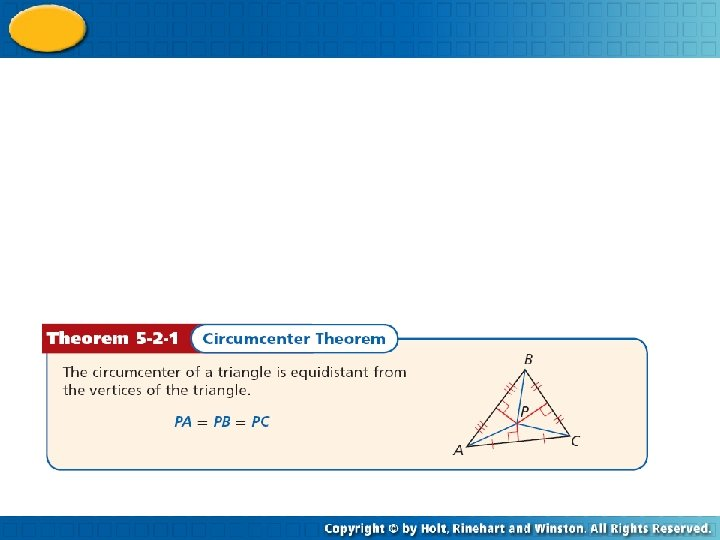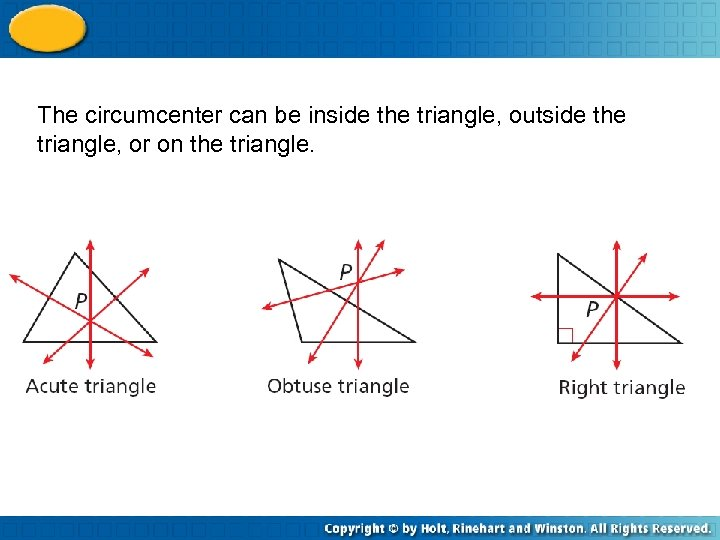The circumcenter can be inside the triangle, outside the triangle, or on the triangle.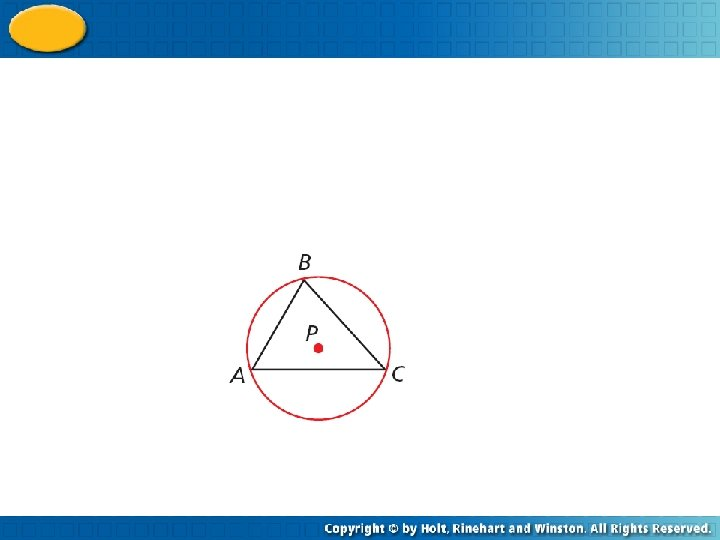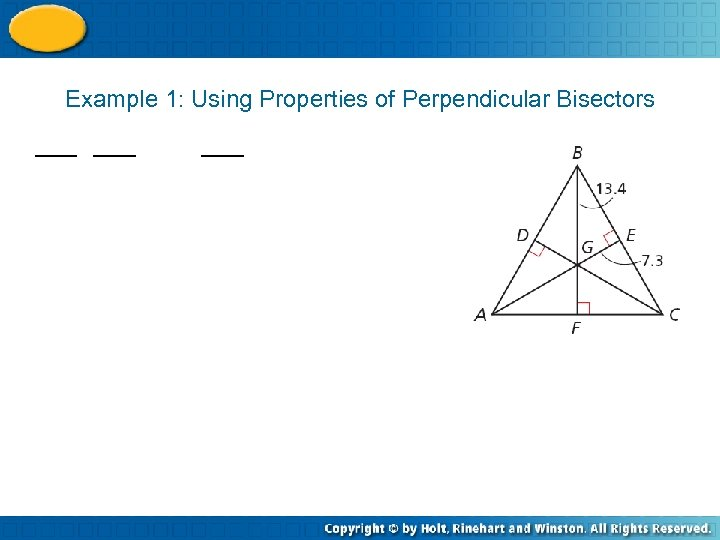Example 1: Using Properties of Perpendicular Bisectors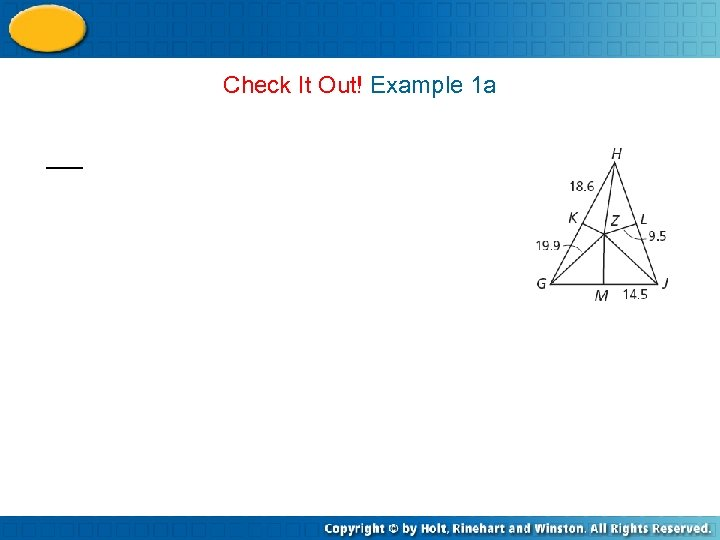Check It Out! Example 1 a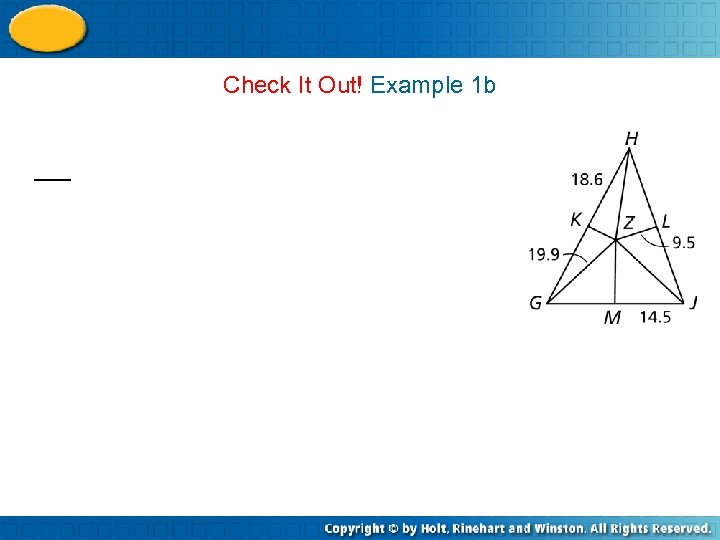Check It Out! Example 1 b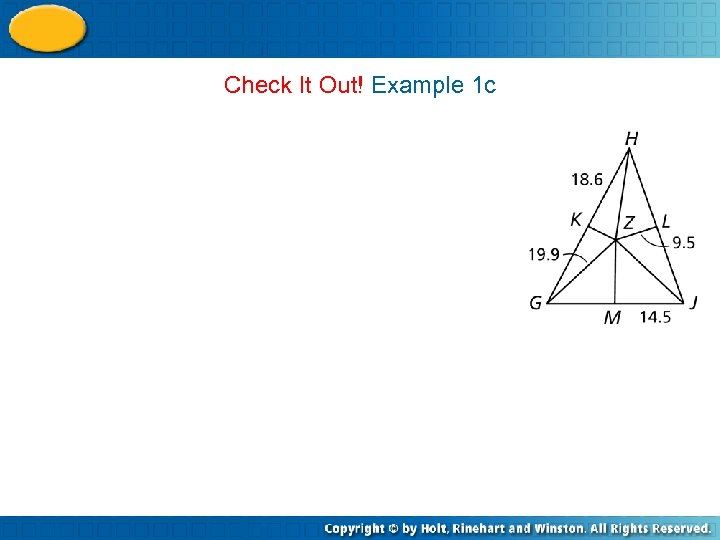Check It Out! Example 1 c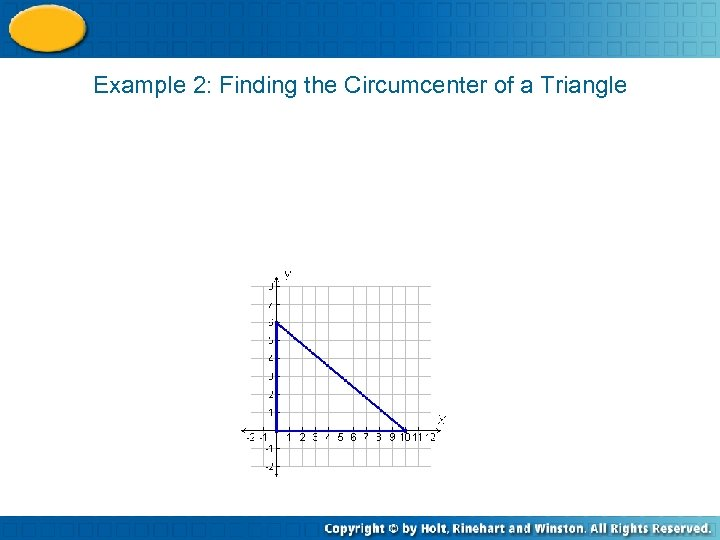Example 2: Finding the Circumcenter of a Triangle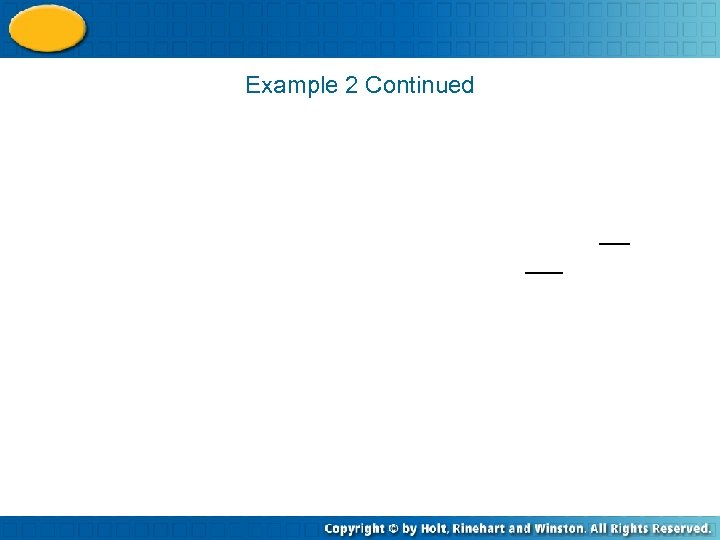Example 2 Continued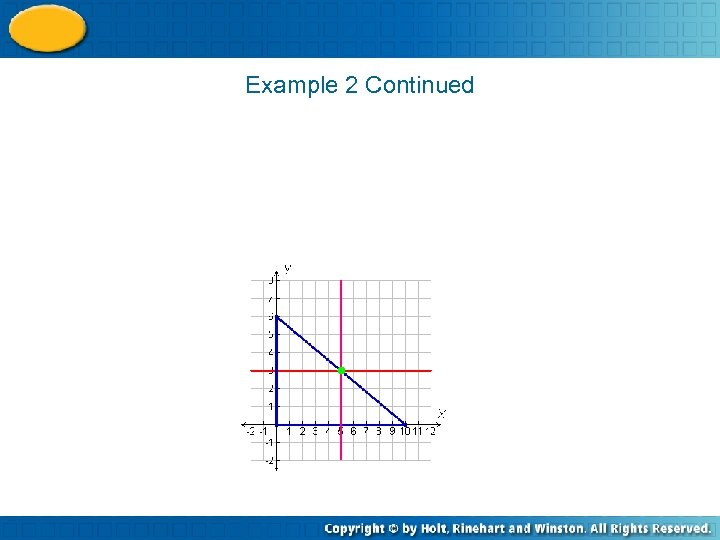Example 2 Continued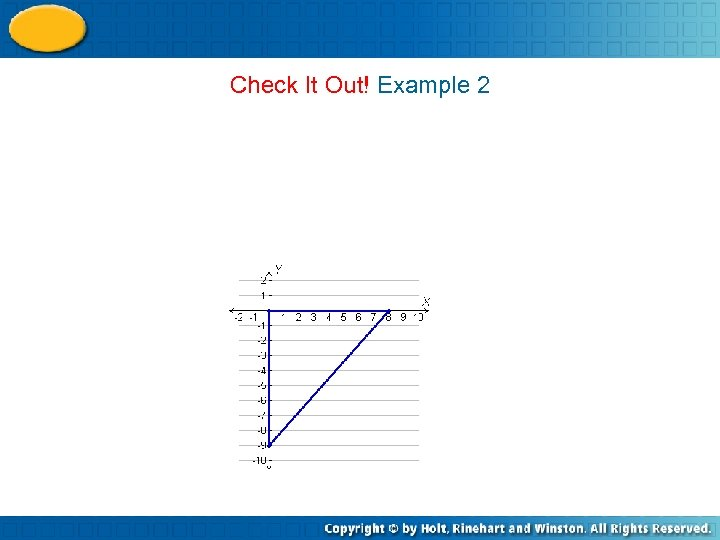Check It Out! Example 2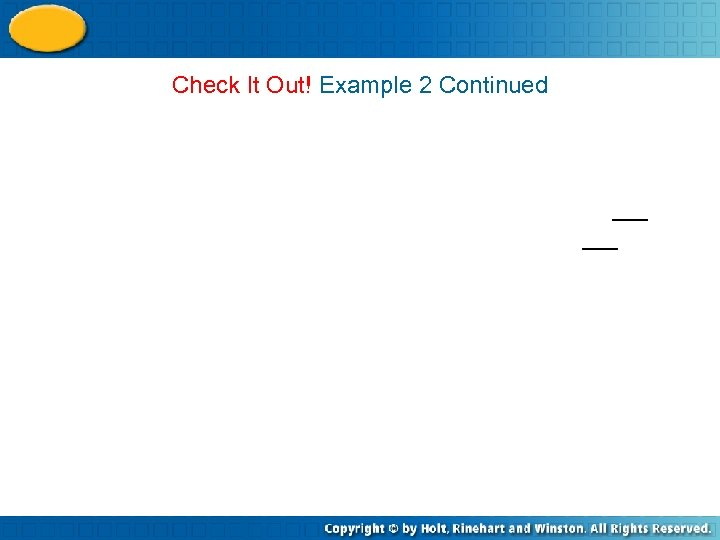Check It Out! Example 2 Continued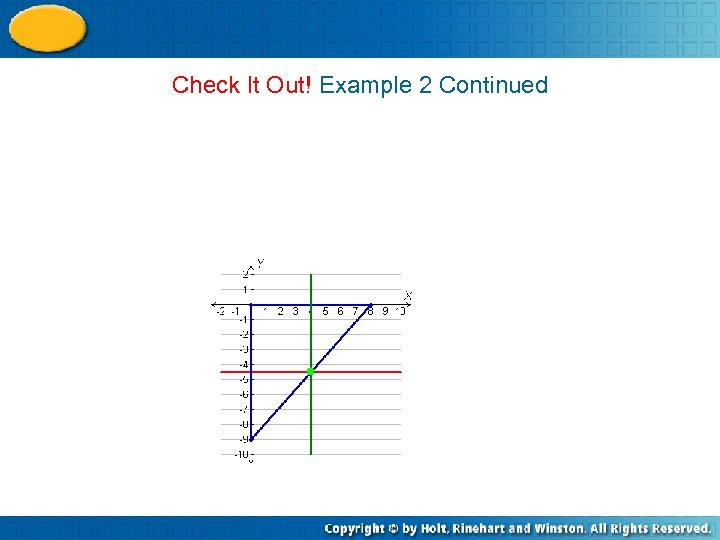Check It Out! Example 2 Continued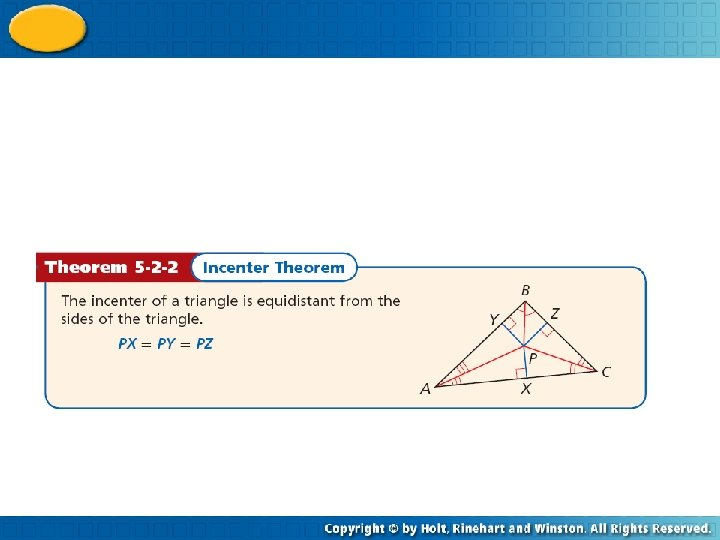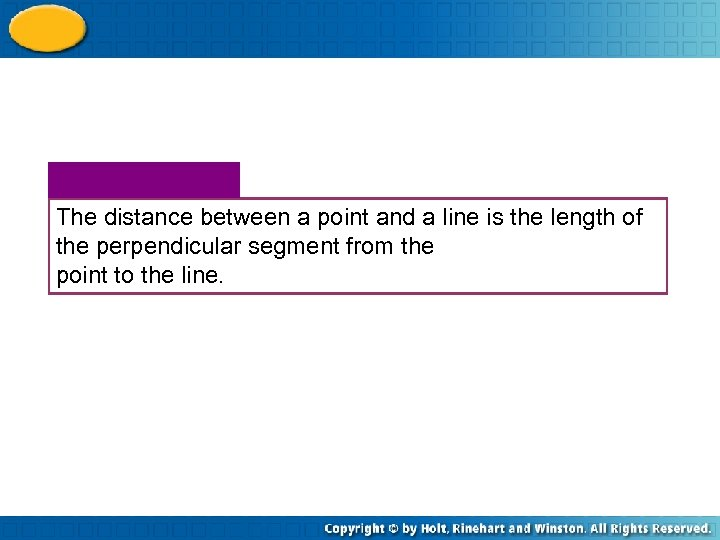The distance between a point and a line is the length of the perpendicular segment from the point to the line.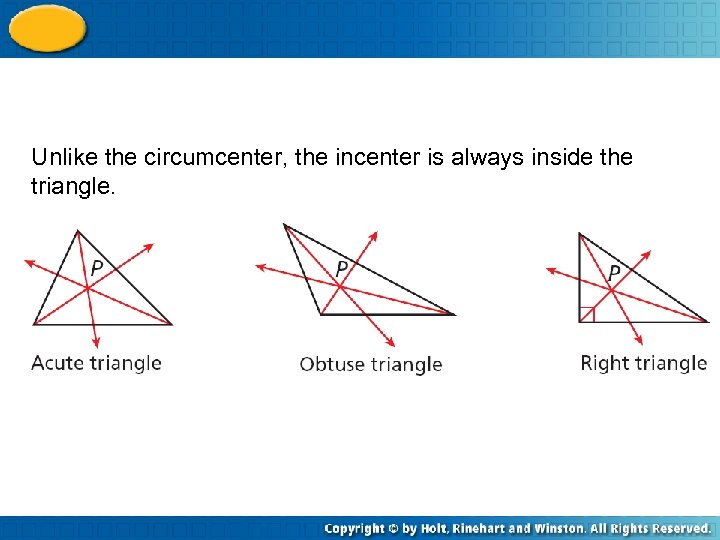Unlike the circumcenter, the incenter is always inside the triangle.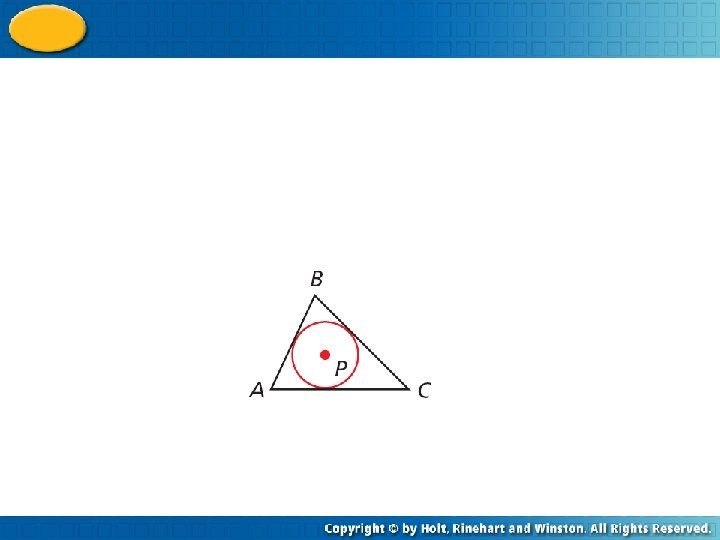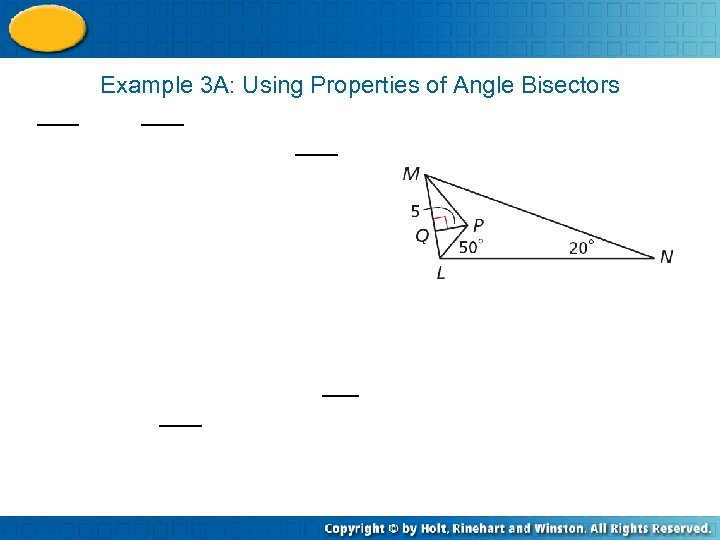Example 3 A: Using Properties of Angle Bisectors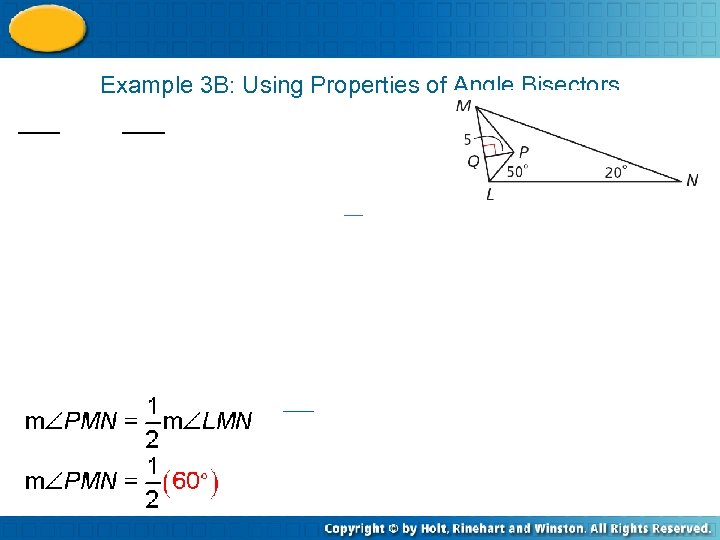Example 3 B: Using Properties of Angle Bisectors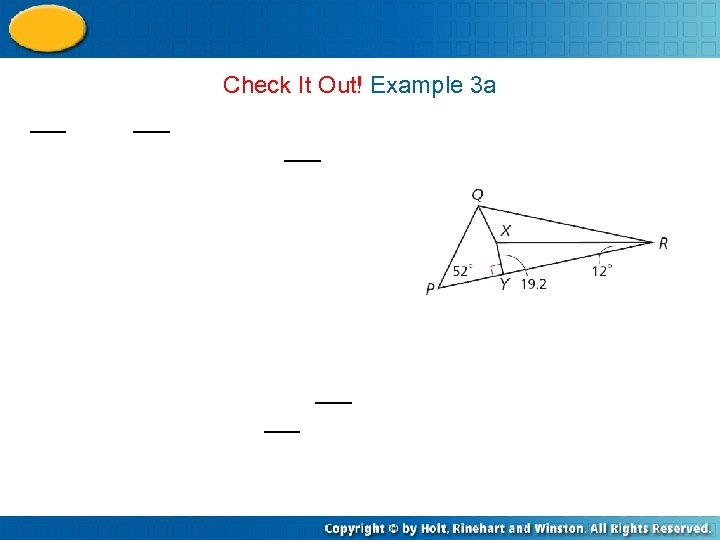Check It Out! Example 3 aCheck It Out! Example 3 b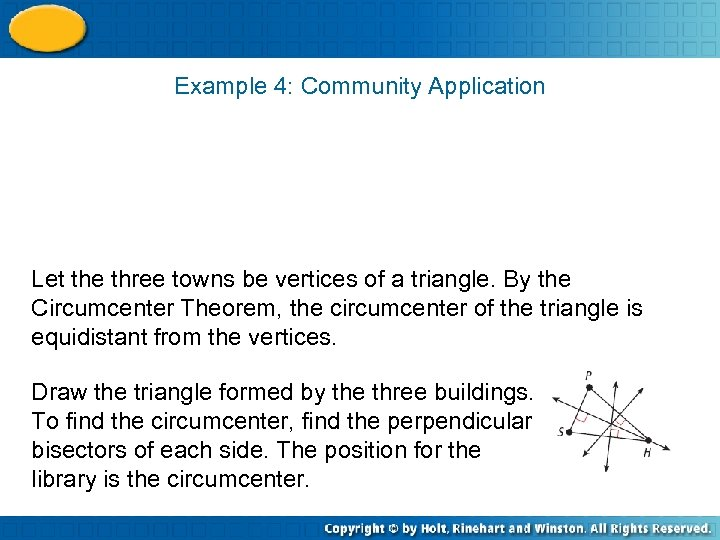Example 4: Community Application Let the three towns be vertices of a triangle. By the Circumcenter Theorem, the circumcenter of the triangle is equidistant from the vertices. Draw the triangle formed by the three buildings. To find the circumcenter, find the perpendicular bisectors of each side. The position for the library is the circumcenter.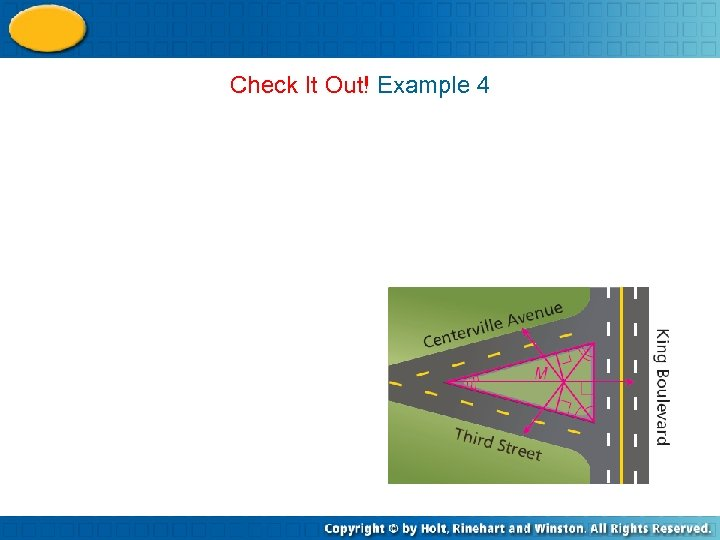Check It Out! Example 4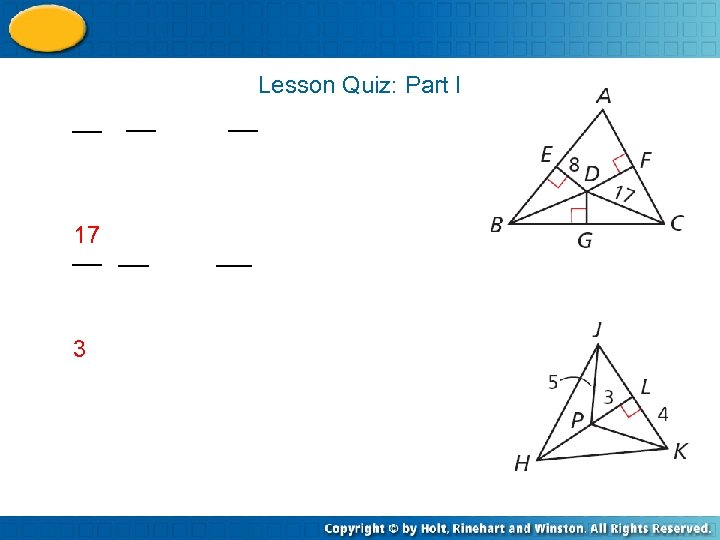Lesson Quiz: Part I 17 3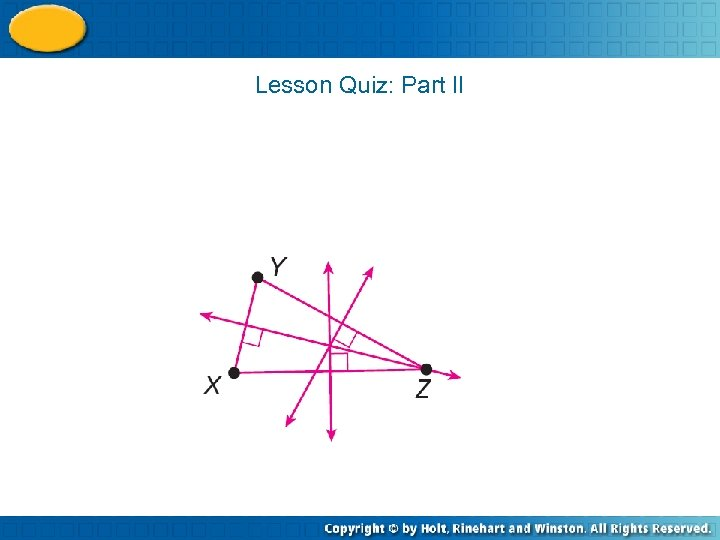Lesson Quiz: Part II